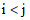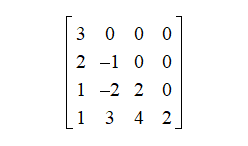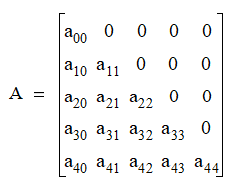# NMath User's Guide

17.1 Lower Triangular Matrices (.NET, C#, CSharp, VB, Visual Basic, F#)

A lower triangular matrix is a square matrix with all elements above the main diagonal equal to zero. That is,for. For example, this is a 4 x 4 lower triangular matrix:Lower triangular matrices often arise at an intermediate stage in solving systems of equations and inverting matrices.

NMath provides lower triangular matrix classes for four datatypes: single- and double-precision floating point numbers, and single- and double-precision complex numbers. The classnames are , , , and .

For efficiency, zero elements above the main diagonal are not stored. Instead, matrix values are stored in a vector row by row. For example, the following 5 x 5 lower triangular matrix:is stored in a data vector as:

```v = [ a00 a10 a11 a20 a21 a22 a30 a31 a32 a33 a40 a41 a42 a43 a44 ]
```

In general, the relationship between matrix and vector indices is:

```A[i,j] = v[i(i+1)/2 + j]
```

Top

Top